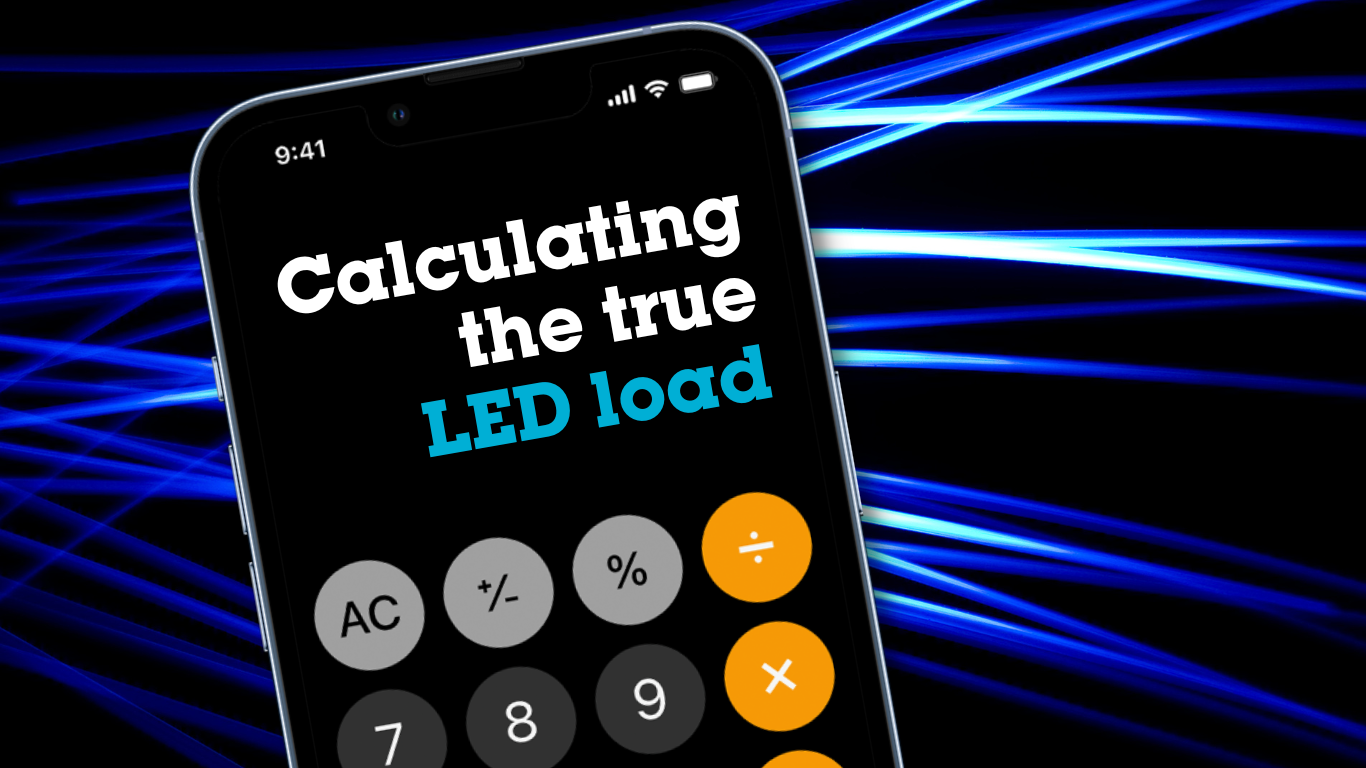# Calculating the true LED load

Have you ever calculated an LED dimming installation, only to become frustrated when the circuit overloads, the dimmer fails, or the fittings, lamps or controls on the circuit don’t work as expected?

These are some of the hallmark signs that the numbers are off, and the load you are trying to control is a lot higher than you had initially thought.

At Zano Controls, our technical team spend a lot of time testing LED lamps, fittings and drivers, and we’ve got decades worth of knowledge in the problems and challenges that arise when pairing LED with lighting control.

So, we’ve created the ultimate guide for you, to assist in your wattage calculations.

## Key terms

Before getting started on the calculations, here are a few things relating to LED lamps, fittings and drivers, and some key definitions you need to consider before purchasing your lighting products.

LED box wattage

We refer to box watts as the number written on an LED lamp or fitting’s packaging.

It’s a contentious subject, but did you know that the wattage published on an LEDs box is very rarely an accurate description of the fitting or lamps total load?

LED drivers

LED driver ratings are rated at the amount of energy they can deliver, not the energy being drawn on the circuit, so the efficiency of the driver needs to be considered when calculating the total load of your install.

Power factor

In an AC circuit, power factor is the ratio between the “real power” and the “reactive power” of a load. The power factor can then be used with the wattage to give us the “apparent power” of the load. This figure can then be used to give us an indication on how in phase the current is with the voltage. This is important when controlling LED lighting as a lower power factor means a higher peak current.

Inrush current

Inrush current is an effect that is caused by capacitance in an LED driver when a driver is first turned on. The capacitors appear almost like a short circuit taking a large amount of current until they are fully charged. Therefore, when you look at the current waveform when switching on an LED load you would see a large spike and then a falling slow curve as the capacitor has charged.

P (Watts) = I (RMS Current) x V (RMS Volts)

The above formula gives you the total load if the power factor is unity and the load is purely resistive. However, LED loads are complex loads, so when you factor in box watts, driver efficiency and power factor you still must consider how the LED driver is actually drawing its current. And this can be completely non-sinusoidal. This means that an RMS, or average current figure, is just not accurate enough, as the current drawn can be “spiky”.

## How to calculate an LED installations’ total load

So, now you know some of the key things to be aware of, we’ll explain how to take all the information above and use it to your advantage to calculate the total load of an install.

Scenario 1) LED lamps or fittings below 10W/VA

Box watts / Power factor = Apparent power

Then take the apparent power and add 10%

We have found that this apparent power figure plus 10% can be used to select a dimmer up to 250W/VA - the 10% allows for the variations in load current as described above.

Scenario 2) LED lamps or fittings below 50W/VA

Box watts / Power factor = Apparent power

Then take the apparent power and add 100% (or double that figure)

We have found that this apparent power figure plus 100% can be used to select a dimmer up to 250W/VA. The 100% allows for the variations in load current. As described above, these variations tend to be worse when dealing with lamps above 10W/VA.

If you have a project where the specification requires using multiple fittings, it would be worth having the fittings tested to ensure the best results.

Scenario 3) LED separate drivers or LED tape drivers up to 50W/VA

N.B. LED drivers above 10W/VA tend to exhibit challenging characteristics, as mentioned above, so the formula below is a guide only.

Box watts / Power factor = Apparent power

Apparent power / Driver efficiency (taken from a driver’s datasheet)

Then take the apparent power and add 100%

We have found that this apparent power figure plus 100% can be used and we would only recommend using our ZBARLED remote dimming packs to dim this type of load as the complexity of the current waveform has proven to cause issues.

If you have a project using multiples of this type of fitting, we recommend contacting us so that we can test and advise on what dimming products would work best with the fittings you have chosen.

Scenario 4) LED separate drivers or LED tape drivers up to 300W/VA

N.B. LED drivers above 50W/VA tend exhibit the worst characteristics. These types of drivers are very difficult to define using a mathematical formula as it involves understanding how the driver has been designed and from that understanding how the current is drawn.

We would recommend contacting us and arranging for us to carry out testing of the fittings/drivers to ascertain the actual characteristics of the load you are trying to dim.